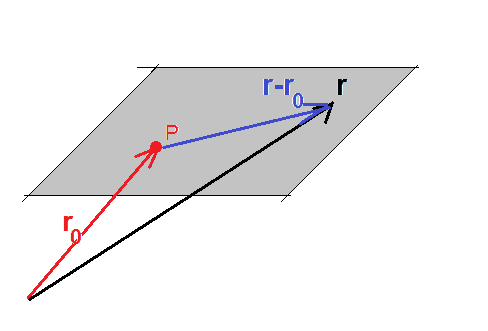# Find the equation of a plane perpendicular to a line and goes through a point

## Homework Statement

find equation of plane P that is perpendicular to line L which passes through the point (-2,-2,3)

...

## The Attempt at a Solution

[/B]line L passes through the points (1,2,1) and (0,0,-3) I have worked out the parametric equations of line L to be
x=1-t
y=2-2t
z=1-4t
i know that if dot product of two vectors = 0 then they are perpendicular if that helps. im stuck on how to piece this question together.
any help would be greatly appreciated

## Answers and Replies

haruspex
Science Advisor
Homework Helper
Gold Member
2020 Award
if dot product of two vectors = 0 then they are perpendicular
Right, so you need a vector in the direction of the line.

•cathal84
Right, so you need a vector in the direction of the line.
i found a directional vector on the line it is <-1,-2,-4> so if i do dot product of that vector with <1,-1/2,0> (i just came up with in my head thinking what vector do i need to have that will give me dot product equal to 0) this will give me the dot product = 0.
so can i conclude that the vector <1,-1/2,0> is on the plane?

i found a directional vector on the line it is <-1,-2,-4> so if i do dot product of that vector with <1,-1/2,0> (i just came up with in my head thinking what vector do i need to have that will give me dot product equal to 0) this will give me the dot product = 0.
so can i conclude that the vector <1,-1/2,0> is on the plane?
even if so not sure how this is going to help me, exam
i found a directional vector on the line it is <-1,-2,-4> so if i do dot product of that vector with <1,-1/2,0> (i just came up with in my head thinking what vector do i need to have that will give me dot product equal to 0) this will give me the dot product = 0.
so can i conclude that the vector <1,-1/2,0> is on the plane?
even if that is true i dont know how that helps me

ehild
Homework Helperif ##\vec r## is a position vector of the plane than the difference ##\vec r - \vec r_0 ## is a vector lying in the plane. It is perpendicular to L. Write it out in components.

haruspex
Science Advisor
Homework Helper
Gold Member
2020 Award
i found a directional vector on the line it is <-1,-2,-4> so if i do dot product of that vector with <1,-1/2,0> (i just came up with in my head thinking what vector do i need to have that will give me dot product equal to 0) this will give me the dot product = 0.
so can i conclude that the vector <1,-1/2,0> is on the plane?
You have to be careful to distinguish between a vector representing a direction of interest and a vector representing a point. If the plane does not pass through the origin then the vector for a point in the plane is not parallel to the plane. Vectors perpendicular to the given line will be parallel to the plane. See ehild's diagram.

member 545369
Alternatively:

You know that the line L is perpendicular to the plane. Also, you found that <-1,-2,-4> is a vector in the same direction as the line. Wouldn't that imply that <-1,-2,-4> is normal to the plane? Knowing the general formula of a plane ##ax+by+cz=d## , what do the coefficients ##a, b, ##& ##c## have to do with the normal to the plane?